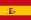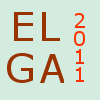ELGA 2011Topics

Topics

The following is a list of the topics of interest for the Workshop.

1. Algebraic curves and their moduli

2. Vector bundles and their moduli

3. Surfaces and higher dimensional varieties

4. Classical projective geometry. Intersection theory and enumerative geometry

5. D-modules and differential algebra

6. Kahler geometry and generalizations. Hodge theory

7. Complex dynamics and holomorphic foliations

8. Automorphic forms, theta functions and modular forms

9. Algebraic groups

10. Arithmetic Algebraic Geometry. Elliptic curves. Abelian varieties

11. Singularity theory

12. Computational methods in algebraic geometry and commutative algebra

13. Connections with combinatorics. Toric varieties. Tropical algebraic geometry

14. Connections with theoretical physics. Mirror symmetry

15. Applications of algebraic geometry to other sciences

Last updated : June 2011